# RD Sharma Solutions For Class 12 Maths Exercise 3.2 Chapter 3 Binary Operations

RD Sharma Solutions Class 12 Maths Exercise 3.2 Chapter 3 Binary Operations  is provided here for students to study and score good marks in their board exams. This exercise deals with types of binary operations. Exercise 3.2 of Chapter 3 consists of problems based on commutativity and associativity of binary operations. Students can refer to the solutions as a major study material to improve their speed in solving problems accurately.

The RD Sharma Solutions prepared by our faculty are in an interactive manner to make it easy for the students to understand the concepts. Students can access RD Sharma Solutions Class 12 Maths Chapter 3 Binary Operations Exercise 3.2 free PDF, which are given here.

## Download the PDF of RD Sharma Solutions For Class 12 Maths Chapter 3 – Binary Operations Exercise 3.2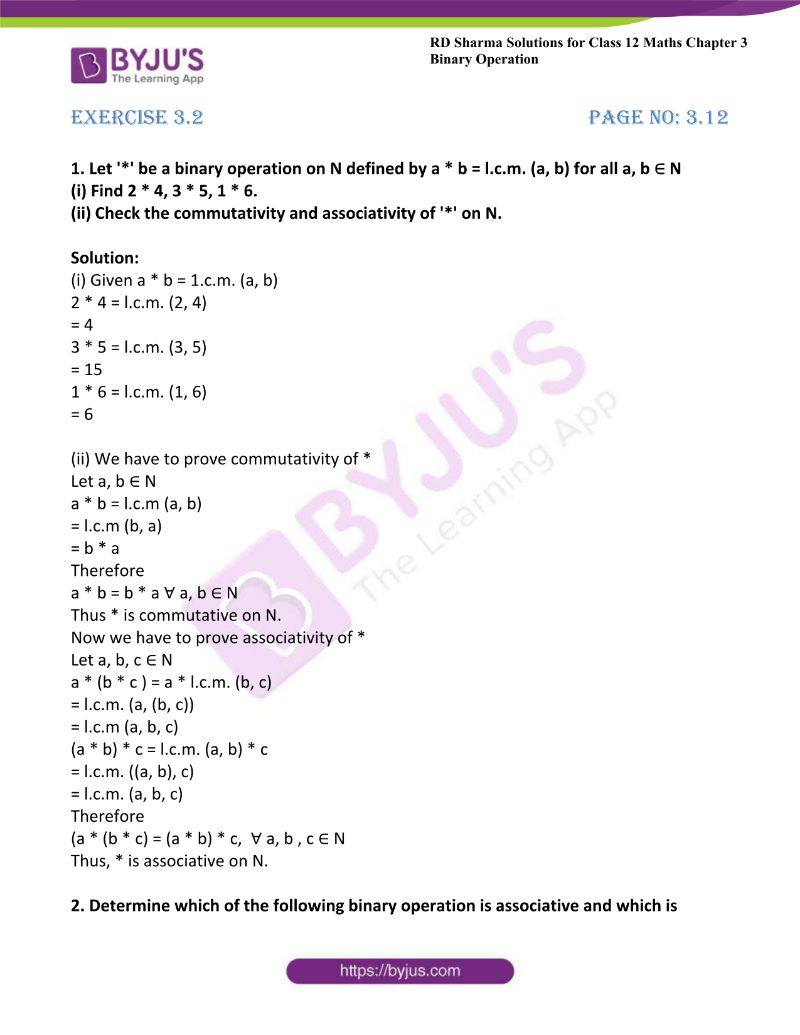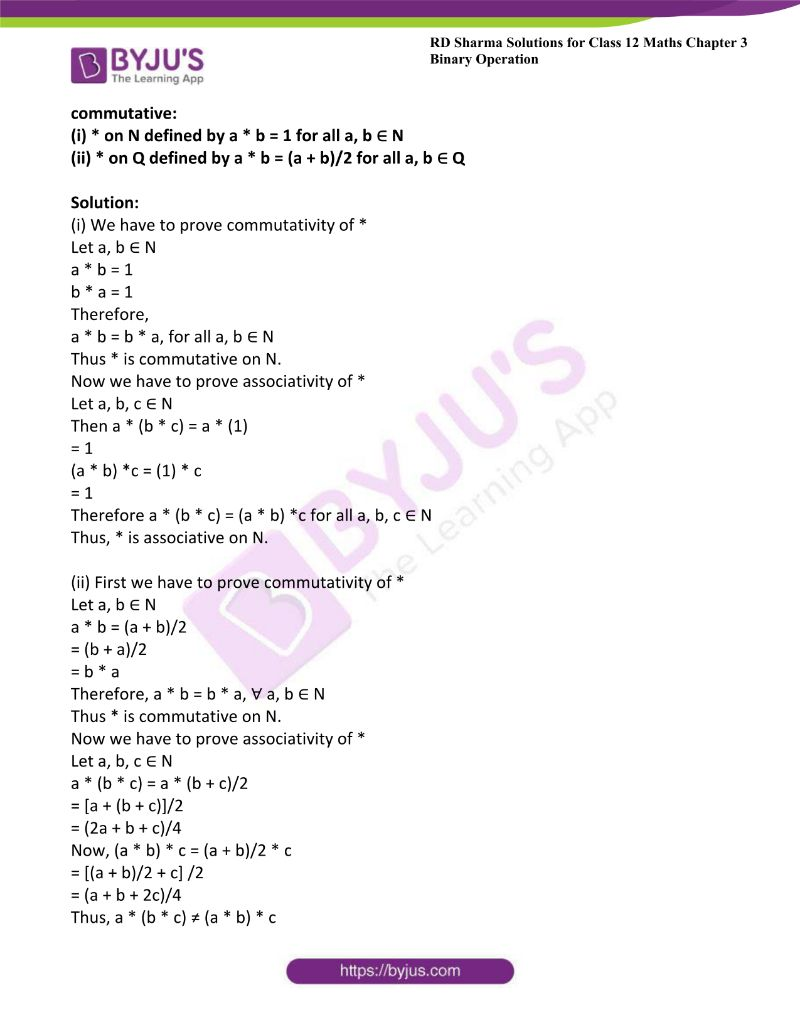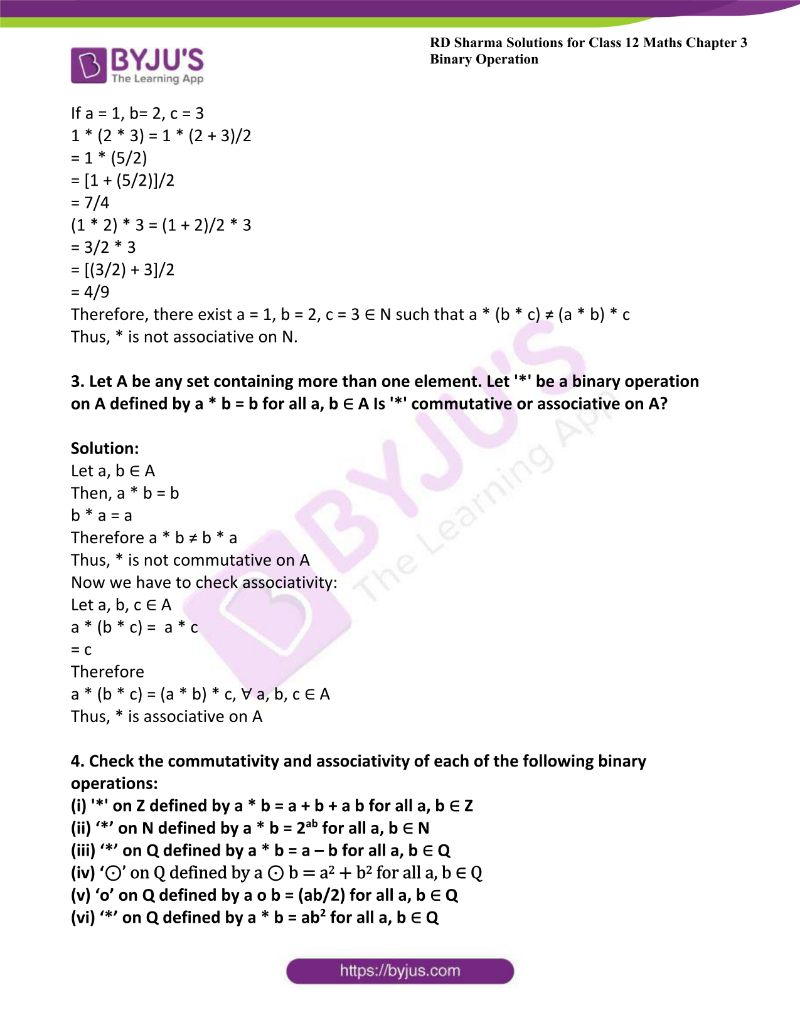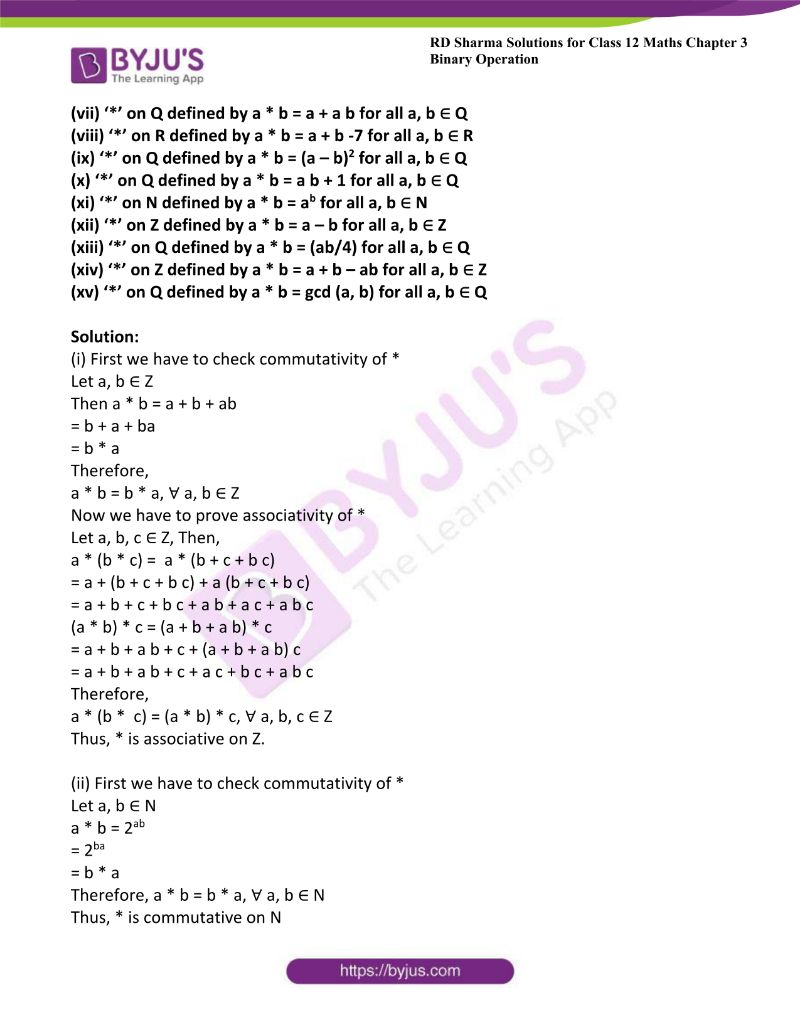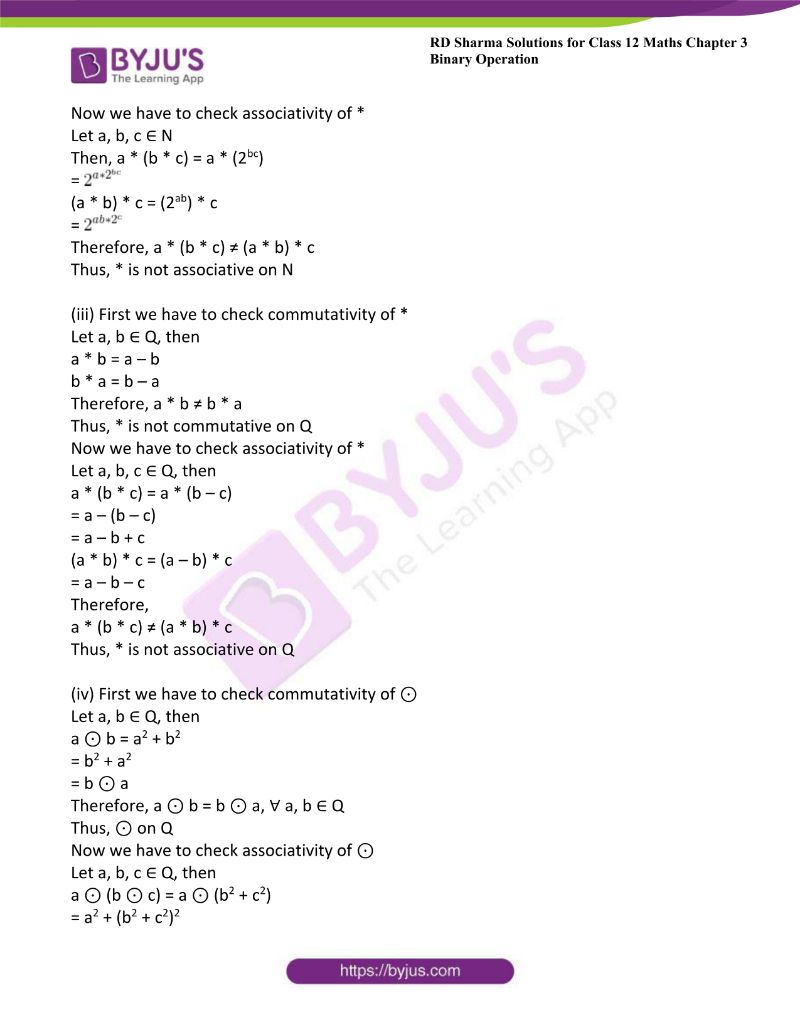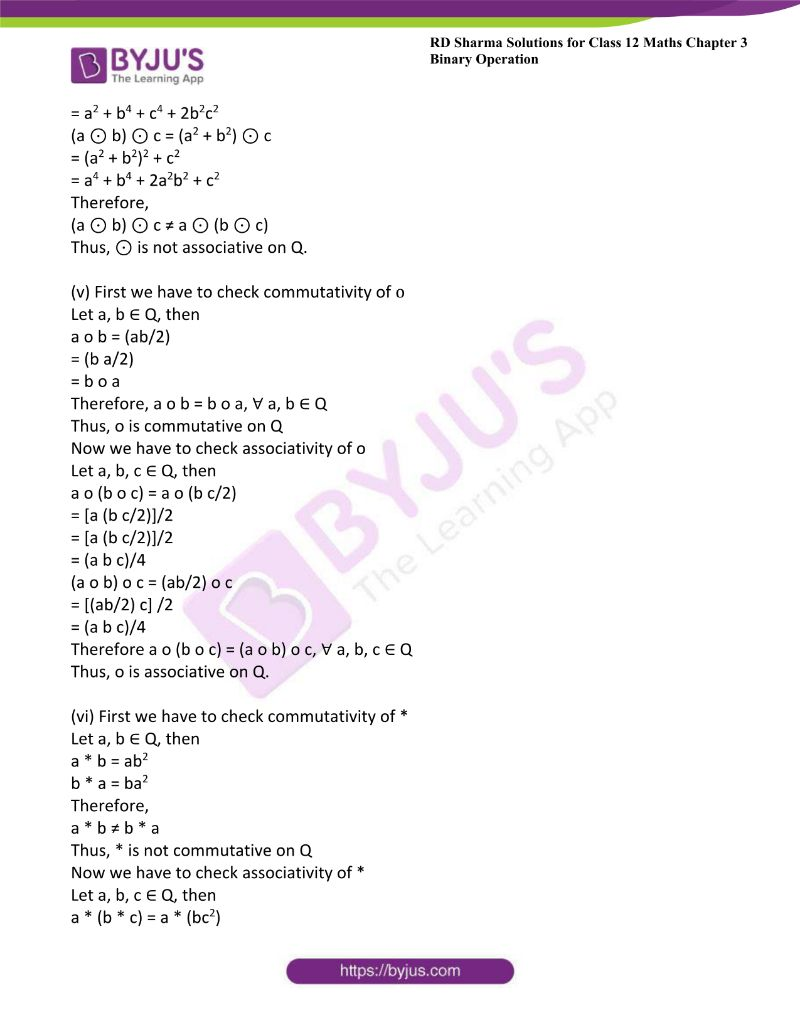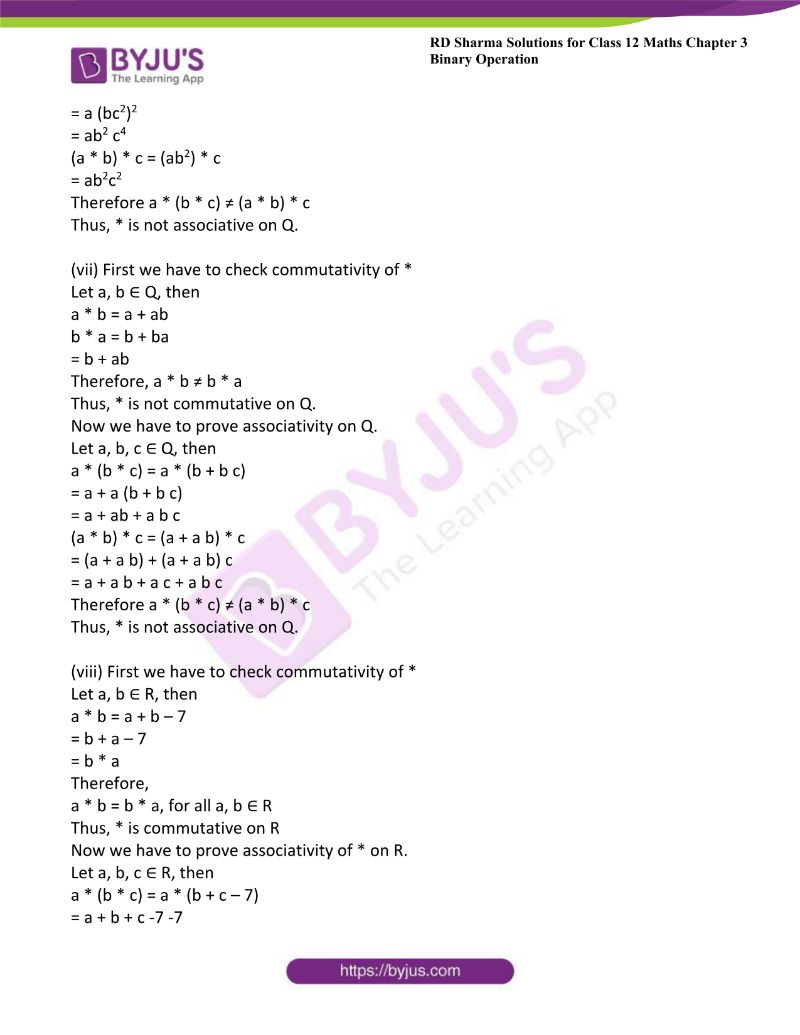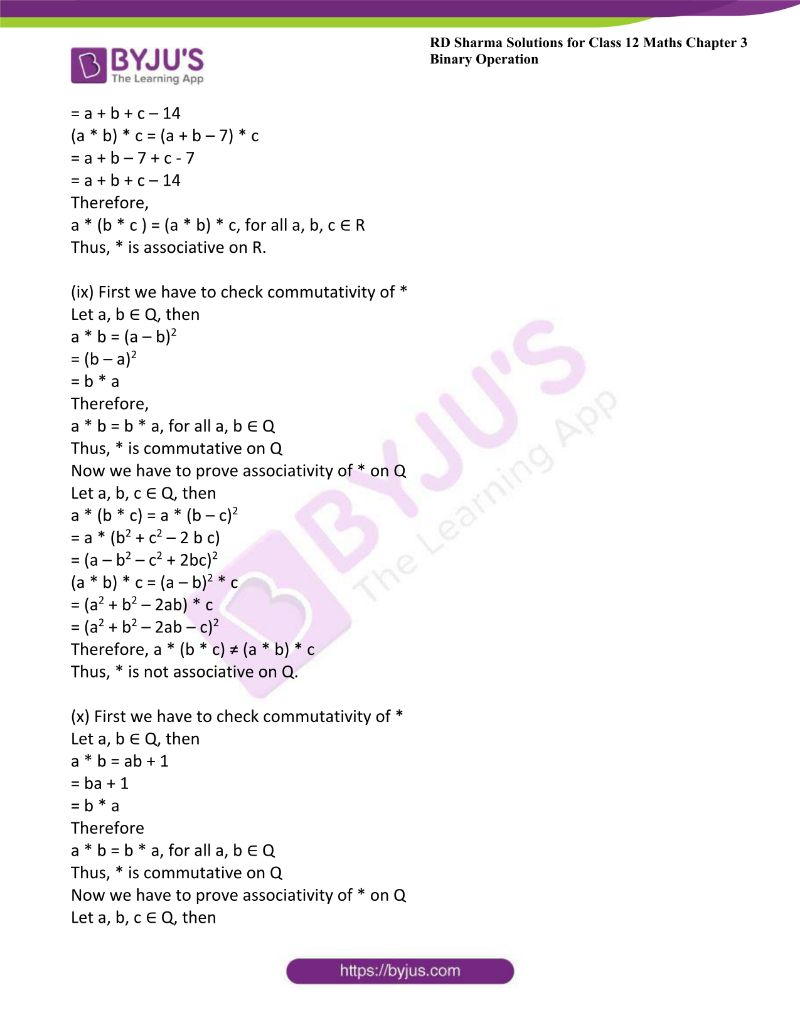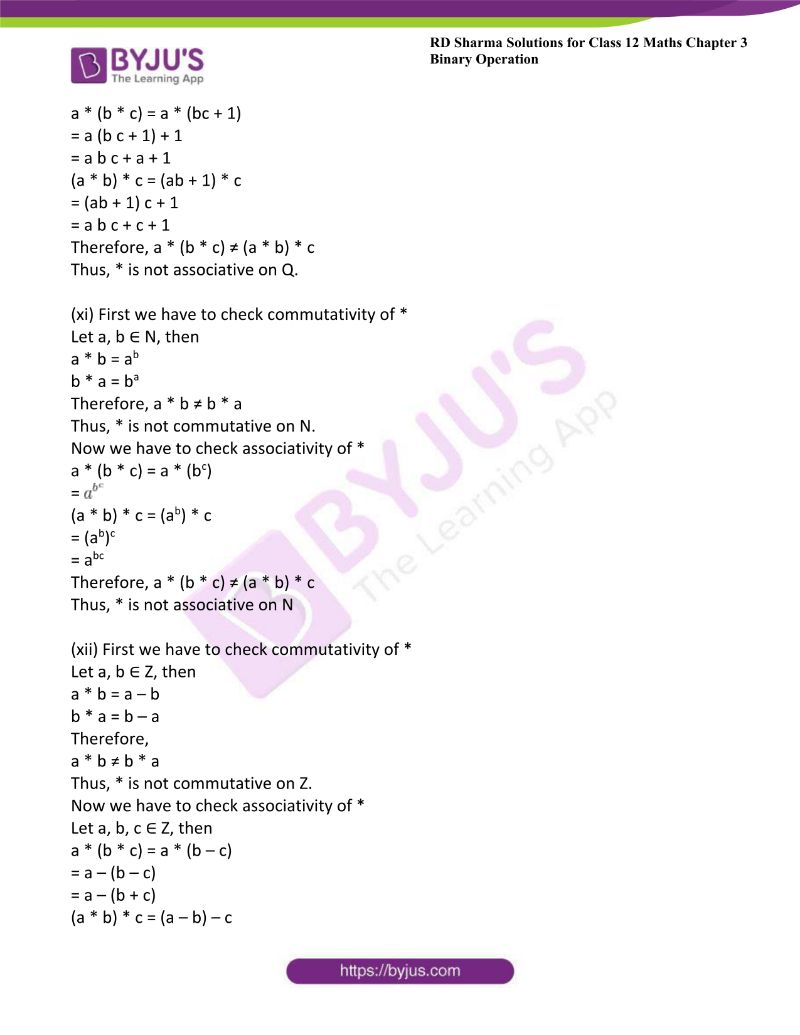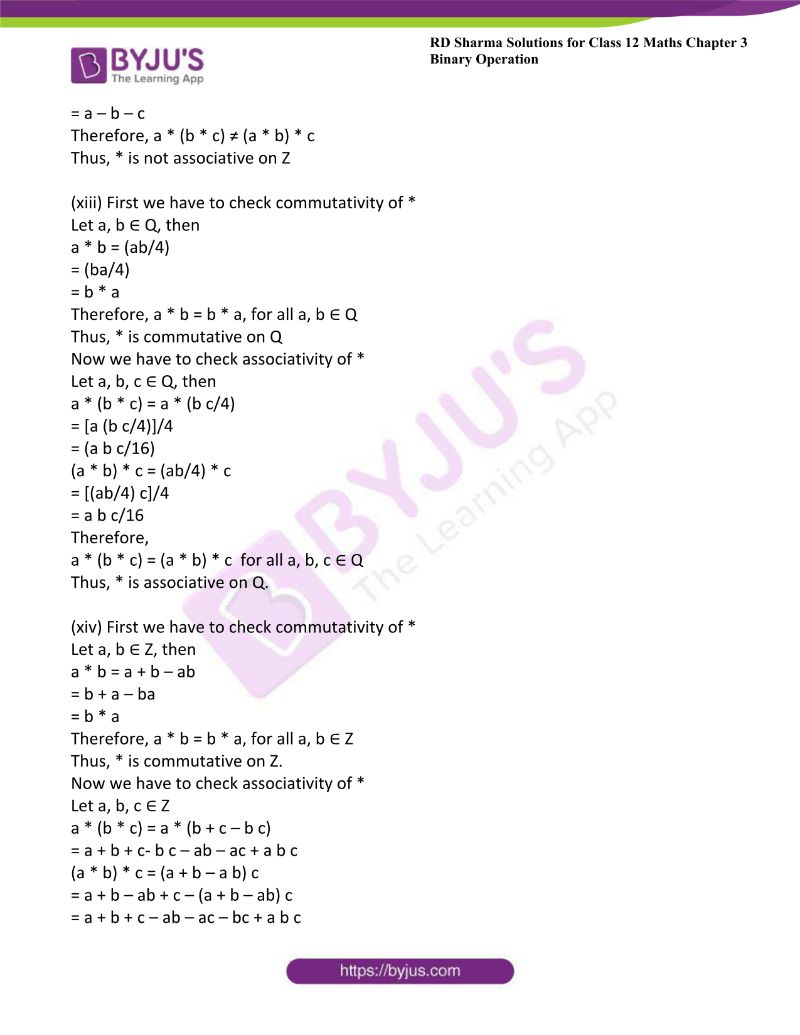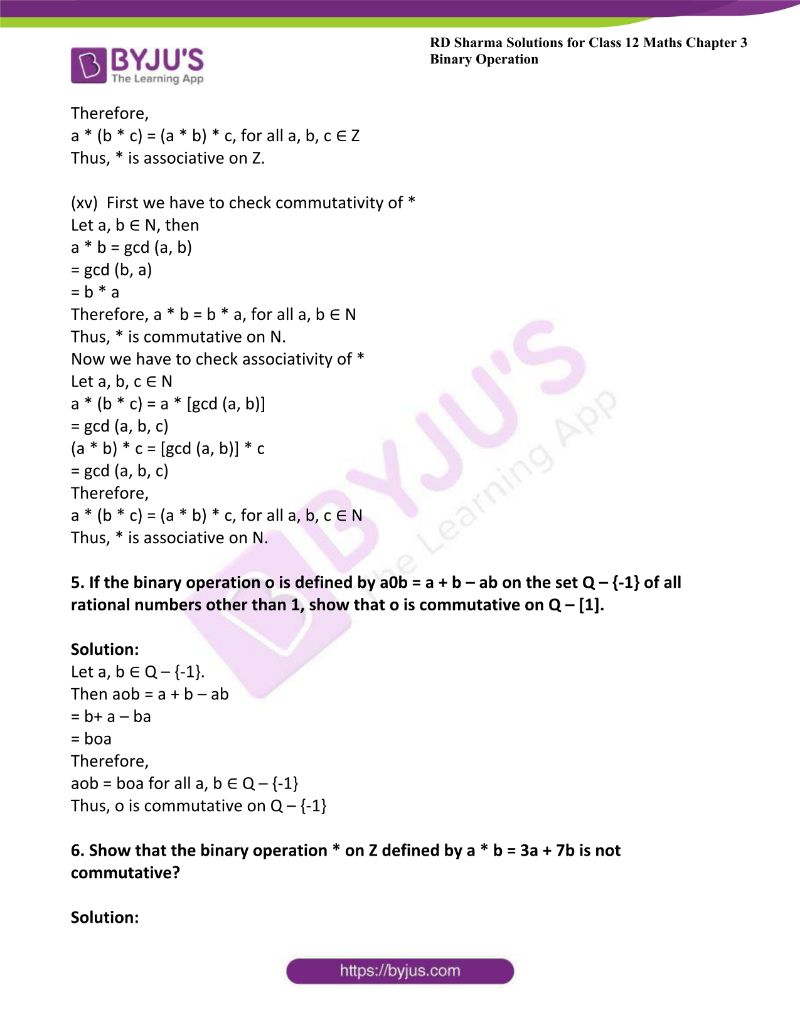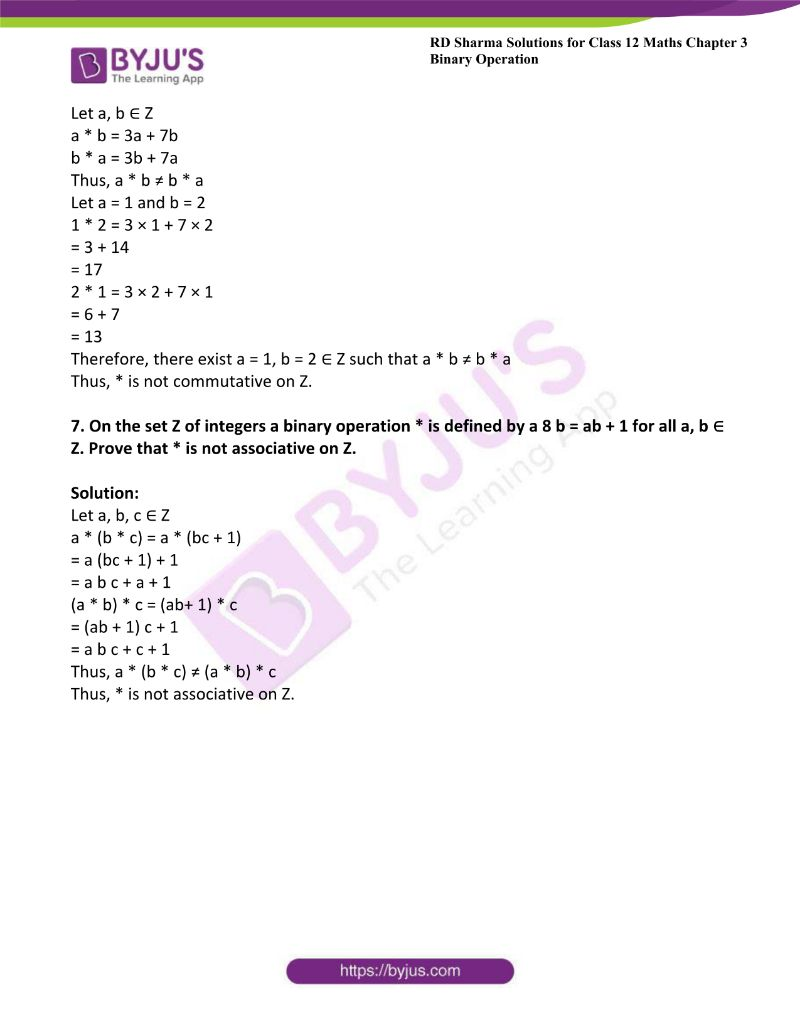### Access Other Exercises of RD Sharma Solutions For Class 12 Maths Chapter 3 – Binary Operations

Exercise 3.1 Solutions

Exercise 3.3 Solutions

Exercise 3.4 Solutions

Exercise 3.5 Solutions

### Access RD Sharma Solutions For Class 12 Maths Chapter 3 – Function Exercise 3.2

1. Let ‘*’ be a binary operation on N defined by a * b = l.c.m. (a, b) for all a, b ∈ N
(i) Find 2 * 4, 3 * 5, 1 * 6.

(ii) Check the commutativity and associativity of ‘*’ on N.

Solution:

(i) Given a * b = 1.c.m. (a, b)

2 * 4 = l.c.m. (2, 4)

= 4

3 * 5 = l.c.m. (3, 5)

= 15

1 * 6 = l.c.m. (1, 6)

= 6

(ii) We have to prove commutativity of *

Let a, b ∈ N

a * b = l.c.m (a, b)

= l.c.m (b, a)

= b * a

Therefore

a * b = b * a ∀ a, b ∈ N

Thus * is commutative on N.

Now we have to prove associativity of *

Let a, b, c ∈ N

a * (b * c ) = a * l.c.m. (b, c)

= l.c.m. (a, (b, c))

= l.c.m (a, b, c)

(a * b) * c = l.c.m. (a, b) * c

= l.c.m. ((a, b), c)

= l.c.m. (a, b, c)

Therefore

(a * (b * c) = (a * b) * c, ∀ a, b , c ∈ N

Thus, * is associative on N.

2. Determine which of the following binary operation is associative and which is commutative:

(i) * on N defined by a * b = 1 for all a, b ∈ N

(ii) * on Q defined by a * b = (a + b)/2 for all a, b ∈ Q

Solution:

(i) We have to prove commutativity of *

Let a, b ∈ N

a * b = 1

b * a = 1

Therefore,

a * b = b * a, for all a, b ∈ N

Thus * is commutative on N.

Now we have to prove associativity of *

Let a, b, c ∈ N

Then a * (b * c) = a * (1)

= 1

(a * b) *c = (1) * c

= 1

Therefore a * (b * c) = (a * b) *c for all a, b, c ∈ N

Thus, * is associative on N.

(ii) First we have to prove commutativity of *

Let a, b ∈ N

a * b = (a + b)/2

= (b + a)/2

= b * a

Therefore, a * b = b * a, ∀ a, b ∈ N

Thus * is commutative on N.

Now we have to prove associativity of *

Let a, b, c ∈ N

a * (b * c) = a * (b + c)/2

= [a + (b + c)]/2

= (2a + b + c)/4

Now, (a * b) * c = (a + b)/2 * c

= [(a + b)/2 + c] /2

= (a + b + 2c)/4

Thus, a * (b * c) ≠ (a * b) * c

If a = 1, b= 2, c = 3

1 * (2 * 3) = 1 * (2 + 3)/2

= 1 * (5/2)

= [1 + (5/2)]/2

= 7/4

(1 * 2) * 3 = (1 + 2)/2 * 3

= 3/2 * 3

= [(3/2) + 3]/2

= 4/9

Therefore, there exist a = 1, b = 2, c = 3 ∈ N such that a * (b * c) ≠ (a * b) * c

Thus, * is not associative on N.

3. Let A be any set containing more than one element. Let ‘*’ be a binary operation on A defined by a * b = b for all a, b ∈ A Is ‘*’ commutative or associative on A?

Solution:

Let a, b ∈ A

Then, a * b = b

b * a = a

Therefore a * b ≠ b * a

Thus, * is not commutative on A

Now we have to check associativity:

Let a, b, c ∈ A

a * (b * c) = a * c

= c

Therefore

a * (b * c) = (a * b) * c, ∀ a, b, c ∈ A

Thus, * is associative on A

4. Check the commutativity and associativity of each of the following binary operations:

(i) ‘*’ on Z defined by a * b = a + b + a b for all a, b ∈ Z

(ii) ‘*’ on N defined by a * b = 2ab for all a, b ∈ N

(iii) ‘*’ on Q defined by a * b = a – b for all a, b ∈ Q

(iv) ‘⊙’ on Q defined by a ⊙ b = a2 + b2 for all a, b ∈ Q

(v) ‘o’ on Q defined by a o b = (ab/2) for all a, b ∈ Q

(vi) ‘*’ on Q defined by a * b = ab2 for all a, b ∈ Q

(vii) ‘*’ on Q defined by a * b = a + a b for all a, b ∈ Q

(viii) ‘*’ on R defined by a * b = a + b -7 for all a, b ∈ R

(ix) ‘*’ on Q defined by a * b = (a – b)2 for all a, b ∈ Q

(x) ‘*’ on Q defined by a * b = a b + 1 for all a, b ∈ Q

(xi) ‘*’ on N defined by a * b = ab for all a, b ∈ N

(xii) ‘*’ on Z defined by a * b = a – b for all a, b ∈ Z

(xiii) ‘*’ on Q defined by a * b = (ab/4) for all a, b ∈ Q

(xiv) ‘*’ on Z defined by a * b = a + b – ab for all a, b ∈ Z

(xv) ‘*’ on Q defined by a * b = gcd (a, b) for all a, b ∈ Q

Solution:

(i) First we have to check commutativity of *

Let a, b ∈ Z

Then a * b = a + b + ab

= b + a + ba

= b * a

Therefore,

a * b = b * a, ∀ a, b ∈ Z

Now we have to prove associativity of *

Let a, b, c ∈ Z, Then,

a * (b * c) = a * (b + c + b c)

= a + (b + c + b c) + a (b + c + b c)

= a + b + c + b c + a b + a c + a b c

(a * b) * c = (a + b + a b) * c

= a + b + a b + c + (a + b + a b) c

= a + b + a b + c + a c + b c + a b c

Therefore,

a * (b * c) = (a * b) * c, ∀ a, b, c ∈ Z

Thus, * is associative on Z.

(ii) First we have to check commutativity of *

Let a, b ∈ N

a * b = 2ab

= 2ba

= b * a

Therefore, a * b = b * a, ∀ a, b ∈ N

Thus, * is commutative on N

Now we have to check associativity of *

Let a, b, c ∈ N

Then, a * (b * c) = a * (2bc)

=

$$\begin{array}{l}2^{a*2^{bc}}\end{array}$$

(a * b) * c = (2ab) * c

=

$$\begin{array}{l}2^{ab*2^{c}}\end{array}$$

Therefore, a * (b * c) ≠ (a * b) * c

Thus, * is not associative on N

(iii) First we have to check commutativity of *

Let a, b ∈ Q, then

a * b = a – b

b * a = b – a

Therefore, a * b ≠ b * a

Thus, * is not commutative on Q

Now we have to check associativity of *

Let a, b, c ∈ Q, then

a * (b * c) = a * (b – c)

= a – (b – c)

= a – b + c

(a * b) * c = (a – b) * c

= a – b – c

Therefore,

a * (b * c) ≠ (a * b) * c

Thus, * is not associative on Q

(iv) First we have to check commutativity of ⊙

Let a, b ∈ Q, then

a ⊙ b = a2 + b2

= b2 + a2

= b ⊙ a

Therefore, a ⊙ b = b ⊙ a, ∀ a, b ∈ Q

Thus, ⊙ on Q

Now we have to check associativity of ⊙

Let a, b, c ∈ Q, then

a ⊙ (b ⊙ c) = a ⊙ (b2 + c2)

= a2 + (b2 + c2)2

= a2 + b4 + c4 + 2b2c2

(a ⊙ b) ⊙ c = (a2 + b2) ⊙ c

= (a2 + b2)2 + c2

= a4 + b4 + 2a2b2 + c2

Therefore,

(a ⊙ b) ⊙ c ≠ a ⊙ (b ⊙ c)

Thus, ⊙ is not associative on Q.

(v) First we have to check commutativity of o

Let a, b ∈ Q, then

a o b = (ab/2)

= (b a/2)

= b o a

Therefore, a o b = b o a, ∀ a, b ∈ Q

Thus, o is commutative on Q

Now we have to check associativity of o

Let a, b, c ∈ Q, then

a o (b o c) = a o (b c/2)

= [a (b c/2)]/2

= [a (b c/2)]/2

= (a b c)/4

(a o b) o c = (ab/2) o c

= [(ab/2) c] /2

= (a b c)/4

Therefore a o (b o c) = (a o b) o c, ∀ a, b, c ∈ Q

Thus, o is associative on Q.

(vi) First we have to check commutativity of *

Let a, b ∈ Q, then

a * b = ab2

b * a = ba2

Therefore,

a * b ≠ b * a

Thus, * is not commutative on Q

Now we have to check associativity of *

Let a, b, c ∈ Q, then

a * (b * c) = a * (bc2)

= a (bc2)2

= ab2 c4

(a * b) * c = (ab2) * c

= ab2c2

Therefore a * (b * c) ≠ (a * b) * c

Thus, * is not associative on Q.

(vii) First we have to check commutativity of *

Let a, b ∈ Q, then

a * b = a + ab

b * a = b + ba

= b + ab

Therefore, a * b ≠ b * a

Thus, * is not commutative on Q.

Now we have to prove associativity on Q.

Let a, b, c ∈ Q, then

a * (b * c) = a * (b + b c)

= a + a (b + b c)

= a + ab + a b c

(a * b) * c = (a + a b) * c

= (a + a b) + (a + a b) c

= a + a b + a c + a b c

Therefore a * (b * c) ≠ (a * b) * c

Thus, * is not associative on Q.

(viii) First we have to check commutativity of *

Let a, b ∈ R, then

a * b = a + b – 7

= b + a – 7

= b * a

Therefore,

a * b = b * a, for all a, b ∈ R

Thus, * is commutative on R

Now we have to prove associativity of * on R.

Let a, b, c ∈ R, then

a * (b * c) = a * (b + c – 7)

= a + b + c -7 -7

= a + b + c – 14

(a * b) * c = (a + b – 7) * c

= a + b – 7 + c – 7

= a + b + c – 14

Therefore,

a * (b * c ) = (a * b) * c, for all a, b, c ∈ R

Thus, * is associative on R.

(ix) First we have to check commutativity of *

Let a, b ∈ Q, then

a * b = (a – b)2

= (b – a)2

= b * a

Therefore,

a * b = b * a, for all a, b ∈ Q

Thus, * is commutative on Q

Now we have to prove associativity of * on Q

Let a, b, c ∈ Q, then

a * (b * c) = a * (b – c)2

= a * (b2 + c2 – 2 b c)

= (a – b2 – c2 + 2bc)2

(a * b) * c = (a – b)2 * c

= (a2 + b2 – 2ab) * c

= (a2 + b2 – 2ab – c)2

Therefore, a * (b * c) ≠ (a * b) * c

Thus, * is not associative on Q.

(x) First we have to check commutativity of *

Let a, b ∈ Q, then

a * b = ab + 1

= ba + 1

= b * a

Therefore

a * b = b * a, for all a, b ∈ Q

Thus, * is commutative on Q

Now we have to prove associativity of * on Q

Let a, b, c ∈ Q, then

a * (b * c) = a * (bc + 1)

= a (b c + 1) + 1

= a b c + a + 1

(a * b) * c = (ab + 1) * c

= (ab + 1) c + 1

= a b c + c + 1

Therefore, a * (b * c) ≠ (a * b) * c

Thus, * is not associative on Q.

(xi) First we have to check commutativity of *

Let a, b ∈ N, then

a * b = ab

b * a = ba

Therefore, a * b ≠ b * a

Thus, * is not commutative on N.

Now we have to check associativity of *

a * (b * c) = a * (bc)

=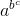(a * b) * c = (ab) * c

= (ab)c

= abc

Therefore, a * (b * c) ≠ (a * b) * c

Thus, * is not associative on N

(xii) First we have to check commutativity of *

Let a, b ∈ Z, then

a * b = a – b

b * a = b – a

Therefore,

a * b ≠ b * a

Thus, * is not commutative on Z.

Now we have to check associativity of *

Let a, b, c ∈ Z, then

a * (b * c) = a * (b – c)

= a – (b – c)

= a – (b + c)

(a * b) * c = (a – b) – c

= a – b – c

Therefore, a * (b * c) ≠ (a * b) * c

Thus, * is not associative on Z

(xiii) First we have to check commutativity of *

Let a, b ∈ Q, then

a * b = (ab/4)

= (ba/4)

= b * a

Therefore, a * b = b * a, for all a, b ∈ Q

Thus, * is commutative on Q

Now we have to check associativity of *

Let a, b, c ∈ Q, then

a * (b * c) = a * (b c/4)

= [a (b c/4)]/4

= (a b c/16)

(a * b) * c = (ab/4) * c

= [(ab/4) c]/4

= a b c/16

Therefore,

a * (b * c) = (a * b) * c for all a, b, c ∈ Q

Thus, * is associative on Q.

(xiv) First we have to check commutativity of *

Let a, b ∈ Z, then

a * b = a + b – ab

= b + a – ba

= b * a

Therefore, a * b = b * a, for all a, b ∈ Z

Thus, * is commutative on Z.

Now we have to check associativity of *

Let a, b, c ∈ Z

a * (b * c) = a * (b + c – b c)

= a + b + c- b c – ab – ac + a b c

(a * b) * c = (a + b – a b) c

= a + b – ab + c – (a + b – ab) c

= a + b + c – ab – ac – bc + a b c

Therefore,

a * (b * c) = (a * b) * c, for all a, b, c ∈ Z

Thus, * is associative on Z.

(xv) First we have to check commutativity of *

Let a, b ∈ N, then

a * b = gcd (a, b)

= gcd (b, a)

= b * a

Therefore, a * b = b * a, for all a, b ∈ N

Thus, * is commutative on N.

Now we have to check associativity of *

Let a, b, c ∈ N

a * (b * c) = a * [gcd (a, b)]

= gcd (a, b, c)

(a * b) * c = [gcd (a, b)] * c

= gcd (a, b, c)

Therefore,

a * (b * c) = (a * b) * c, for all a, b, c ∈ N

Thus, * is associative on N.

5. If the binary operation o is defined by a0b = a + b – ab on the set Q – {-1} of all rational numbers other than 1, show that o is commutative on Q – .

Solution:

Let a, b ∈ Q – {-1}.

Then aob = a + b – ab

= b+ a – ba

= boa

Therefore,

aob = boa for all a, b ∈ Q – {-1}

Thus, o is commutative on Q – {-1}

6. Show that the binary operation * on Z defined by a * b = 3a + 7b is not commutative?

Solution:

Let a, b ∈ Z

a * b = 3a + 7b

b * a = 3b + 7a

Thus, a * b ≠ b * a

Let a = 1 and b = 2

1 * 2 = 3 × 1 + 7 × 2

= 3 + 14

= 17

2 * 1 = 3 × 2 + 7 × 1

= 6 + 7

= 13

Therefore, there exist a = 1, b = 2 ∈ Z such that a * b ≠ b * a

Thus, * is not commutative on Z.

7. On the set Z of integers a binary operation * is defined by a 8 b = ab + 1 for all a, b ∈ Z. Prove that * is not associative on Z.

Solution:

Let a, b, c ∈ Z

a * (b * c) = a * (bc + 1)

= a (bc + 1) + 1

= a b c + a + 1

(a * b) * c = (ab+ 1) * c

= (ab + 1) c + 1

= a b c + c + 1

Thus, a * (b * c) ≠ (a * b) * c

Thus, * is not associative on Z.